# FAN_COMPONENT

Specifies a fan component for an element set.

AcuSolve Command

## Syntax

FAN_COMPONENT("name") {parameters...}

User-given name.

## Parameters

shape (enumerated) [no default]
Shape of the surfaces.
three_node_triangle or tri3
Three-node triangle.
six_node_triangle or tri6
Six-node triangle.
element_set or elem_set (string) [no default]
User-given name of the parent element set. This element set is turned into a fan component by this command.
surfaces (array) [no default]
List of component inlet element surfaces.
surface_sets (list) [={}]
List of surface set names (strings) to use in this fan component. When using this option, the connectivity, shape, and parent element of the surfaces are provided by the surface set container and it is unnecessary to specify the shape, element_set and surfaces parameters directly to the FAN_COMPONENT command. This option is used in place of directly specifying these parameters. In the event that both the surface_sets and surfaces parameters are provided, the full collection of surface elements is read and a warning message is issued. The surface_sets option is the preferred method to specify the surface elements. This option provides support for mixed element topologies and simplifies pre-processing and post-processing.
type (enumerated) [=axial]
Type of fan.
axial
Axial fan. Fluid enters axially.
center direction [={0,0,0}]
A point on the center of rotation, specified in the global xyz coordinate system.
direction or dir (array) [={1,0,0}]
Axial direction of the fan, specified in the global xyz coordinate system.
angular_speed or omega (real) [=1]
Fan rotational speed, in radians per unit of time.
angular_speed_multiplier_function (string) [=none]
User-given name of the multiplier function for scaling the angular speed. If none, no scaling is performed.
Radius of the tip of the fan.
thickness (real) >0 [=1]
Fan thickness.
constant (enumerated) [=constant]
Type of the axial coefficient.
constant or const
Constant for the entire set. Requires axial_coefficient.
piecewise_linear or linear
Piecewise bilinear curve fit. Requires axial_curve_fit_values, axial_curve_fit_row_variable and axial_curve_fit_column_variable.
user_function or user
User-defined function. Requires axial_user_function, axial_user_values and axial_user_strings.
axial_coefficient (real) [=1]
The constant value of the axial coefficient. Used with constant.
axial_curve_fit_values (array) [={0,0;0,1}]
Axial coefficient as a two dimensional curve fit table with two independent variables. Used with piecewise_linear axial coefficient type.
Independent variable of the rows of the axial curve fit table. Used with piecewise_linear axial coefficient type.
axial_curve_fit_column_variable (enumerated) (=normalized_flow_rate)
Independent variable of the columns of the axial curve fit table. Used with piecewise_linear axial coefficient type.
normalized_flow_rate or flow_rate
Flow rate normalized with respect to inlet area, density, and tip velocity.
axial_user_function (string) [no default]
Name of the user-defined function for the axial coefficient. Used with user_function axial coefficient type.
axial_user_values (array) [={}]
Array of values to be passed to the user-defined function. Used with user_function axial coefficient type.
axial_user_strings (list) [={}]
Array of strings to be passed to the user-defined function. Used with user_function axial coefficient type.
axial_multiplier_function (string) [=none]
User-given name of the multiplier function for scaling the axial coefficient. If none, no scaling is performed.
Type of the radial coefficient
constant or const
Constant for the entire set. Requires radial_coefficient.
piecewise_linear or linear
user_function or user
The constant value of the radial coefficient. Used with constant radial coefficient type.
Radial coefficient as a two dimensional curve fit table with two independent variables. Used with piecewise_linear radial coefficient type.
Independent variable of the rows of the radial curve fit table. Used with piecewise_linear radial coefficient type.
Independent variable of the columns of the radial curve fit table. Used with piecewise_linear radial coefficient type.
normalized_flow_rate or flow_rate
Flow rate normalized with respect to inlet area, density, and tip velocity.
radial_user_function (string) [no default]
Name of the user-defined function for the radial coefficient. Used with user_function radial coefficient type.
Array of values to be passed to the user-defined function. Used with user_function radial coefficient type.
Array of strings to be passed to the user-defined function. Used with user_function radial coefficient type.
User-given name of the multiplier function for scaling the radial coefficient. If none, no scaling is performed.
tangential_coefficient_type (enumerated) [=constant]
Type of the tangential coefficient.
constant or const
Constant for the entire set. Requires tangential_coefficient.
piecewise_linear or linear
Piecewise bilinear curve fit. Requires tangential_curve_fit_values, tangential_curve_fit_row_variable and tangential_curve_fit_column_variable.
user_function or user
User-defined function. Requires tangential_user_function, tangential_user_values and tangential_user_strings.
tangential_coefficient (real) [=1]
The constant value of the tangential coefficient. Used with constant tangential coefficient type.
tangential_curve_fit_values (array) [={0,0;0,1}]
Tangential coefficient as a two dimensional curve fit table with two independent variables. Used with piecewise_linear tangential coefficient type.
Independent variable of the rows of the tangential curve fit table. Used with piecewise_linear tangential coefficient type.
tangential_curve_fit_column_variable (enumerated) [=normalized_flow_rate]
Independent variable of the columns of the tangential curve fit table. Used with piecewise_bilinear tangential coefficient type.
normalized_flow_rate or flow_rate
Flow rate normalized with respect to inlet area, density, and tip velocity.
tangential_user_function (string) [no default]
Name of the user-defined function for the tangential coefficient. Used with user_function tangential coefficient type.
tangential_user_values (array) [={}]
Array of values to be passed to the user-defined function. Used with user_function tangential coefficient type.
tangential_user_strings (list) [={}]
Array of strings to be passed to the user-defined function. Used with user_function tangential coefficient type.
tangential_multiplier_function (string) [=none]
User-given name of the multiplier function for scaling the tangential coefficient. If none, no scaling is performed.
pq_curve_type (enumerated) [=none]
Type of the fan pressure-flow rate (P-Q) performance curve to simplify the fan modeling process. You can specify fan performance directly through P-Q curve, by-passing the legacy approach of non-dimensional coefficient calculations.
none
None. Specified when using non-dimensional coefficient as input.
piecewise_linear

Piecewise linear curve fit. Requires pq_curve_fit_values.

pq_curve_fit_values (array) [={}]
The pq_curve_fit_values parameter is a two-dimensional array which defines the fan performance curve with the first column of flow rate in m3/s and the second column of pressure increase in Pa.
swirl_factor (real) [=0]
Ratio of tangential body force and the primary body force.

## Description

This command specifies the parameters of a fan component. It also turns all the elements of an element set into a single fan.

For example,
ELEMENT_SET( "engine fan" ) {
shape                           = four_node_tet
elements                        = { 1, 1, 9, 8, 3 ;
2, 3, 8, 9, 5 ;... }
...
}
FAN_COMPONENT( "fan" ) {
shape                           = three_node_triangle
element_set                     = "engine fan"
surfaces                        = { 1, 101, 1, 9, 8 ;
2, 102, 8, 9, 5 ; }
type                            = axial
center                          = { 0, 0, 0 }
direction                       = { 1, 0, 0 }
angular_speed                   = 3600 * (2*PI) / 60 # 3600 RPM
thickness                       = 0.06
axial_coefficient_type          = piecewise_bilinear
axial_curve_fit_values          = {
0 ,   0.0876, 0.0943, 0.1098, 0.1523, 0.1724, 0.2632 ;
0.43, 0.3783, 0.2685, 0.2385, 0.1783, 0.1493, 0.0185 ;
0.61, 0.3878, 0.2738, 0.2429, 0.1856, 0.1576, 0.0246 ;
0.72, 0.3925, 0.2782, 0.2482, 0.1909, 0.1649, 0.0289 ;
0.95, 0.3943, 0.2819, 0.2517, 0.1962, 0.1692, 0.0342 ;
axial_curve_fit_column_variable = normalized_flow_rate
tangential_coefficient_tye      = piecewise_bilinear
tangential_curve_fit_values     = {
0 ,   0.0876, 0.0943, 0.1098, 0.1523, 0.1724, 0.2632 ;
0.43, 0.0205, 0.0351, 0.0774, 0.1102, 0.1299, 0.0608 ;
0.61, 0.0899, 0.0764, 0.0771, 0.0798, 0.0766, 0.0423 ;
0.72, 0.0599, 0.0523, 0.0572, 0.0603, 0.0587, 0.0398 ;
0.95, 0.0401, 0.0344, 0.0399, 0.0412, 0.0402, 0.0254 ;
tangential_curve_fit_column_variable   = normalized_flow_rate
}

turns the "engine fan" element set into a fan component. The inlet of this axial fan is defined by the two surfaces (element faces) of elements 1 and 2. The axis of the fan passes through the global point (zero, zero, zero) in the global xyz coordinate system. The fan blows in the positive global x-direction. The fan speed is 3600 RPM (377 radians per second) in the clockwise direction. The fan tip radius (at the shroud) is 0.11. The distance from the front to the rear of the fan, fan thickness, is 0.06. The axial and tangential coefficients of the fan use a two-dimensional curve fit, while the radial coefficient is zero.

There are two main forms of this command. The previous version (or single topology version) of the command relies on the use of the surfaces parameter to define the surfaces. When using this form of the command, all surfaces within a given set must have the same shape, and it is necessary to include both the element_set and shape parameters in the command. shape specifies the shape of the surface. This shape must be compatible with the shape of the "parent" element set whose user-given name is provided by element_set. The element set shape is specified by the shape parameter of the ELEMENT_SET command. The compatible shapes are:
Element shape
Surface shape
four_node_tet
three_node_triangle
five_node_pyramid
three_node_triangle
five_node_pyramid
six_node_wedge
three_node_triangle
six_node_wedge
eight_node_brick
ten_node_tet
six_node_triangle

The surfaces parameter contains the faces of the element set. This parameter is a multi-column array. The number of columns depends on the shape of the surface. For three_node_triangle, this parameter has five columns, corresponding to the element number (of the parent element set), a unique (within this set) surface number, and the three nodes of the element face. For four_node_quad, surfaces has six columns, corresponding to the element number, a surface number, and the four nodes of the element face. For six_node_triangle, surfaces has eight columns, corresponding to the element number, a surface number, and the six nodes of the element face. One row per surface must be given. The three, four, or six nodes of the surface may be in any arbitrary order, since they are reordered internally based on the parent element definition.

The mixed topology form of the FAN_COMPONENT command provides a more powerful and flexible mechanism for defining the surfaces. Using this form of the command, it is possible to define a collection of surfaces that contains different element shapes. This is accomplished through the use of the surface_sets parameter. The element faces are first created in the input file using the SURFACE_SET command, and are then referred to by the FAN_COMPONENT command. For example, a collection of triangular and quadrilateral element faces can be defined using the following SURFACE_SET commands.
SURFACE_SET( "tri faces" ) {
surfaces    = { 1, 1, 1, 2, 4 ;
2, 2, 3, 4, 6 ;
3, 3, 5, 6, 8 ; }
shape       = three_node_triangle
volume_set  = "tetrahedra"
}
SURFACE_SET( "quad faces" ) {
surfaces    = { 1, 1, 1, 2, 4, 9 ;
2, 2, 3, 4, 6, 12 ;
3, 3, 5, 6, 8, 15 ; }
volume_set  = "prisms"
Then, a single FAN_COMPONENT command is defined that contains the tri and quad faces as follows:
FAN_COMPONENT( "fan" ) {
surface_sets       = { "tri_faces", "quad_faces" }
...
}
The list of surface sets can also be placed in a file, such as surface_sets.srfst:
tri faces
quad faces
FAN_COMPONENT( "fan" ) {
surface_sets       = Read ("surface_sets.srfst")
...
}

The mixed topology version of the FAN_COMPONENT command is preferred. This version provides support for multiple element topologies within a single instance of the command and simplifies pre-processing and post-processing. In the event that both of the surface_sets and surfaces parameters are provided in the same instance of the command, the full collection of surface elements is read and a warning message is issued. Although the single and mixed topology formats of the commands can be combined, it is strongly recommended that they are not.

Two types of fans are supported. In an axial type, the fluid enters the fan in the axial direction, such as in the case of an engine fan. In a radial type, the flow enters radially at the hub of the fan, such as in the case of a blower fan.

A fan component is modeled by adding axial, tangential, and radial forces to the momentum equations. For an axial fan type, these forces increase the pressure across the component by: (1)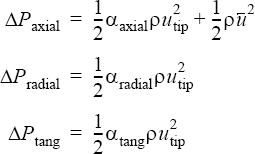where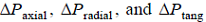are, respectively, the pressure increases in the axial, radial, and tangential directions;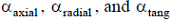are, respectively, the axial, radial, and tangential coefficients of the fan component, specified by axial_coefficient_type, radial_coefficient_type, tangential_coefficient_type, and related parameters; $\rho$ is the density;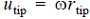is the tip velocity; $\omega$ is the fan rotational speed, specified by angular_speed;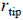is the fan tip radius, specified by tip_radius; andis the mass averaged velocity through the inlet surface of the fan, where the inlet surface is specified by surfaces.

For a radial fan type, the pressure increases in the radial and tangential directions are the same as for an axial type. However, the pressure increase in the axial direction is given by: (2)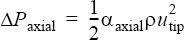The orientation of the fan is defined by radial and direction. These are in the global xyz coordinate system. The fan speed is given by angular_speed. Angular speed is in radians per unit time and uses the right hand rule to define the direction of the fan rotations. For positive values, the fan (looking in the direction axis) rotates clockwise, while for negative values the fan rotates in the counter-clockwise direction.

tip_radius defines the radius at the tip of the fan. This parameter must be consistent with the calibration data used to derive the fan coefficients.

thickness specifies the thickness of the fan. For an axial fan, this is the thickness in the axial direction of the fan. For a radial fan, this is the distance from the hub to the shroud.

A constant axial (tangential or radial) coefficient applies a uniform coefficient value to all quadrature points of the element set. For the above example, the radial coefficient is uniformly set to zero.

A piecewise_bilinear axial coefficient type defines a piecewise bilinear curve fit as a function of two independent variables. axial_curve_fit_row_variable and axial_curve_fit_column_variable define the two independent variables. The axial_curve_fit_values parameter is a two dimensional array which defines the coefficients of the interpolation. The (zero, zero) entry of this array must be zero. The first row of the table defines the values of the independent column variable, while the first column of the table defines the values of the independent row variable. The values of both independent variables must be in ascending order. For the above example, the first row (0.0876, ..., 0.2632) defines the normalized_flow_rate values and the first column (0.43, ..., 0.95) defines the normalized_radius values. The values
0.3783 ... 0.0185
...
0.3943 ... 0.0342

are the axial coefficients.

The coefficients may be read from a file. For the above example, the axial coefficients may be placed in a file, such as fan.axial.fit:
0    0.0876 0.0943 0.1098 0.1523 0.1724 0.2632
0.43 0.3783 0.2685 0.2385 0.1783 0.1493 0.0185
0.61 0.3878 0.2738 0.2429 0.1856 0.1576 0.0246
0.72 0.3925 0.2782 0.2482 0.1909 0.1649 0.0289
0.95 0.3943 0.2819 0.2517 0.1962 0.1692 0.0342
FAN_COMPONENT( "fan" ) {
...
axial_curve_fit_values = Read( "fan.axial.fit" )
}

A piecewise_bilinear tangential or radial coefficient type is defined as in the axial case.

The user_function type for axial, tangential or radial coefficients allows for a more complex behavior; see the AcuSolve User-Defined Functions Guide for a detailed description of user-defined functions.

For example, consider the case where the tangential coefficient is a quadratic function of average inlet velocity and decays linearly with radius.
FAN_COMPONENT( "fan with UDF tangential coefficient" ) {
...
tangential_coefficient_type  = user_function
tangential_user_function     = "usrFanExample"
tangential_user_values       = { 0,   0,  0,    # fan center
1,   0,  0,    # fan direction
0,   0,  0.2,  # velocity coefs
0.11,          # fan tip radius
3600              # fan speed
}
}
where the user-defined function "usrFanExample" may be implemented as follows:
#include "acusim.h"
#include "udf.h"
UDF_PROTOTYPE( usrFanExample ) ; /* function prototype */
Void usrFanExample (
UdfHd             udfHd,                                                      /* Opaque handle for accessing data */
Real*             outVec,                                                     /* Output vector  */
Integer           nItems,                                                     /* Number of elements  */
Integer           vecDim                                                      /* = 1  */
) {
Integer            elem ;                                                      /* an element counter  */
Real               coef0 ;                                                     /* constant coefficient  */
Real               coef1 ;                                                     /* linear coefficient  */
Real               coef2 ;                                                     /* quadratic coefficient */
Real               radRat ;                                                    /* radius ratio */
Real               radius ;                                                    /* fan radius  */
Real               rpm ;                                                       /* fan speed  */
Real               tipVel ;                                                    /* tip velocity  */
Real               tmp ;                                                       /* a temporary value */
Real               velCoef ;                                                   /* velocity coefficient  */
Real               xCenter ;                                                   /* x-coordinates of cente  */
Real               xDir ;                                                      /* x-direction of fan  */
Real               xPnt ;                                                      /* x-coord. of the point   */
Real               yCenter ;                                                   /* y-coordinates of center  */
Real               yDir ;                                                      /* y-direction of fan  */
Real               yPnt ;                                                      /* y-coord. of the point  */
Real               zCenter ;                                                   /* z-coordinates of center  */
Real               zDir ;                                                      /* z-direction of fan  */
Real               zPnt ;                                                      /* z-coord. of the point  */
Real*              aveVel ;                                                    /* average velocity  */
Real*              crd ;                                                       /* coordinates   */
Real*              usrVals ;                                                   /* user values  */
Real*              xCrd ;                                                      /* x-coordinates  */
Real*              yCrd ;                                                      /* y-coordinates  */
Real*              zCrd ;                                                      /* z-coordinates  */
char*              name ;                                                      /* set name   */
udfCheckNumUsrVals( udfHd, 11 ) ;                                                  /* check for error  */
usrVals            = udfGetUsrVals( udfHd ) ;                                  /* get the user vals */
xCenter            = usrVals ;                                              /* get x-center  */
yCenter            = usrVals ;                                              /* get y-center  */
zCenter            = usrVals ;                                              /* get z-center  */
xDir               = usrVals ;                                              /* get x-direction   */
yDir               = usrVals ;                                              /* get y-direction    */
zDir               = usrVals ;                                              /* get z-direction   */
coef0              = usrVals ;                                              /* get const coef.   */
coef1              = usrVals ;                                              /* get lin. coef.    */
coef2              = usrVals ;                                              /* get quad. coef.  */
radius             = usrVals ;                                              /* get fan radius   */
rpm                = usrVals ;                                             /* get fan RPM    */
name               = udfGetName( udfHd ) ;                                     /* get the fan name  */
aveVel             = udfGetFanData( udfHd, name, UDF_FAN_AVERAGE_VELOCITY ) ;  /* get ave. velocity */
crd                = udfGetElmCrd( udfHd ) ;                                   /* get the coord.  */
xCrd               = &crd[0*nItems] ;                                          /* localize x-coord.  */
yCrd               = &crd[1*nItems] ;                                          /* localize y-coord.  */
zCrd               = &crd[2*nItems] ;                                          /* localize z-coord.  */
tipVel             = radius                                                    /* tip velocity  */
* rpm * 2 * 3.1415926535897931 / 60 ;
tmp                = *aveVel / tipVel ;                                        /* velocity ratio  */
velCoef            = coef0 + coef1 * tmp + coef2 * tmp * tmp ;                 /* quadratic func.  */
for ( elem         = 0 ; elem < nItems ; elem++ ) {
xPnt           = xCrd[elem] - xCenter ;
yPnt           = yCrd[elem] - yCenter ;
zPnt           = zCrd[elem] - zCenter ;
tmp            = xDir * xPnt + yDir * yPnt + zDir * zPnt ;
radRat         = xPnt * xPnt + yPnt * yPnt + zPnt * zPnt
- tmp * tmp ;
if ( radRat < 0 ) radRat = 0 ;
outVec[elem]   = velCoef * ( 1. - radRat ) ;
}
} /* end of usrFanExample() */

The dimension of the returned coefficient vector, outVec, is the number of elements.

The angular_speed_multiplier_function, axial_multiplier_function, tangential_multiplier_function, and radial_multiplier_function parameters may be used to scale the angular speed, and the axial, tangential, and radial coefficients respectively. The value of each of these parameters refers to the user-given name of a MULTIPLIER_FUNCTION command in the input file. For example, to impose a uniform axial coefficient as a function of time, the following commands may be issued:
FAN_COMPONENT( "fan" ) {
...
type                          = axial
center                        = { 0, 0, 0 }
direction                     = { 1, 0, 0 }
angular_speed                 = 3600 * (2*PI) / 60 # 3600 RPM
thickness                     = 0.06
axial_coefficient_type        = constant
axial_coefficient             = 1.
axial_multiplier_function     = "time varying"
tangential_coefficient_type   = constant
tangential_coefficient        = 0
}
MULTIPLIER_FUNCTION( "time varying" ) {
type                         = piecewise_linear
curve_fit_values             = { 0, 0.0 ;10, 1.0 ;20, 0.5 ;40, 0.7 ;80, 1.2 ; }
curve_fit_variable           = time
}
AcuSolve 2020 (or a later release) can provide an option of specifying the fan performance curve directly, by-passing the non-dimensional coefficient calculation. As a consequence of this, input variables such as tip_radius and angular_speed are not required. Below is an example:
FAN_COMPONENT( "Fan_Inlet" ) {
surface_sets                        = {  "Fan_Inlet_Fan_Upstream_Duct" }
type                                = axial
center                              = { 0, 0, 0 }
direction                           = { 1, 0, 0 }
thickness                           = 0.06
pq_curve_type                       = piecewise_linear
pq_curve_fit_values                 = {525.35, 494.910;
890.21, 474.63;
1161.63, 424.9;
1272.76, 389.11;
1356.57, 350.42;
1431.84, 308.18;
1494.69, 268.35;
1551.39, 230.89;
}
swirl_factor                        = 0.0
}

The axis of the axial fan passes through the origin of the global coordinate system. The fan blows in the positive x-direction. The distance from the front to the rear of the fan, fan thickness, is 0.06 m. The fan performance curve is defined as the two-dimensional curve fit, while the swirl factor is set to zero. The first column of the table defines the flow rate (m3/s), while the second column of the table is the pressure increase (Pa). The swirl factor is a ratio of the tangential body force to the primary body force (axial or radial).

Some scalar data are written to disk for each fan component and at every time step. This data can be translated to other formats using the AcuTrans program and other post-processing modules; see the AcuSolve Programs Reference Manual for details.